MoveGenerator contains parent classes for all move generators. A MoveGenerator sub-class is used at fullrmc’s stochastic engine runtime to generate moves upon selected groups. Every group has its own MoveGenerator class and definitions, therefore it is possible to fully customize how a group of atoms should move.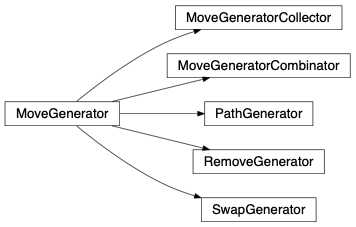class fullrmc.Core.MoveGenerator.MoveGenerator(group=None)

Bases: object

It is the parent class of all moves generators. This class can’t be instantiated but its sub-classes might be.

Parameters: group (None, Group): The group instance.
group

Group instance.

set_group(group)

Set the MoveGenerator group.

Parameters: group (None, Group): Group instance.
check_group(group)

Check the generator’s group. This method must be overloaded in all MoveGenerator sub-classes.

Parameters: group (Group): the Group instance
transform_coordinates(coordinates, argument=None)

Transform coordinates. This method is called to move atoms. This method must be overloaded in all MoveGenerator sub-classes.

Parameters: coordinates (np.ndarray): The coordinates on which to apply the move. argument (object): Any other argument needed to perform the move. In General it’s not needed. coordinates (np.ndarray): The new coordinates after applying the move.
move(coordinates)

Moves coordinates. This method must NOT be overloaded in MoveGenerator sub-classes.

Parameters: coordinates (np.ndarray): The coordinates on which to apply the transformation. coordinates (np.ndarray): The new coordinates after applying the transformation.
class fullrmc.Core.MoveGenerator.RemoveGenerator(group=None, maximumCollected=None, allowFittingScaleFactor=False, atomsList=None)

This is a very particular move generator that will not generate moves on atoms but removes them from the atomic configuration using a general collector mechanism. Remove generators must be used to create defects in the simulated system. When the standard error is high, removing atoms might reduce the total fit standard error but this can be illusional and very limiting because artificial non physical voids can get created in the system which will lead to an impossibility to finding a solution at the end. It’s strongly recommended to exhaust all ideas and possibilities in finding a good solution prior to start removing atoms unless structural defects is the goal of the simulation.

All removed or amputated atoms are collected by the engine and will become available to be re-inserted in the system if needed. But keep in mind, it might be physically easy to remove and atom but an impossibility to add it back especially if the created voids are smeared out.

Removers are called generators but they behave like selectors. Instead of applying a certain move on a group of atoms, they normally pick atoms from defined atoms list and apply no moves on those. ‘move’ and ‘transform_coordinates’ methods are not implemented in this class of generators and a usage error will be raised if called. ‘pick_from_list’ method is used instead and must be overloaded by all RemoveGenerator subclasses.

N.B. This class can’t be instantiated but its sub-classes might be.

Parameters: group (None, Group): The group instance which is this case must be fullrmc EmptyGroup. maximumCollected (None, Integer): The maximum number allowed of atoms to be removed and collected from atomic configuration by the stochastic engine. This property is general to the system and checks engine’s collected atoms not the number of removed atoms via this generator. If None is given, the remover will not check for the number of already removed atoms before attempting a remove. allowFittingScaleFactor (bool): Constraints and especially experimental ones have a scale factor constant that can be fit. Fitting a scale factor happens at stochastic engine’s runtime at a certain fitting frequency. If this flag set to True, then fitting the scale factor will be allowed upon removing atoms. When set to False, fitting the constraint scale factor will be forbidden upon removing atoms. By default, allowFittingScaleFactor is set to False because it’s more logical to allow removing only atoms that enhances the total standard error without rescaling the model’s data. atomsList (None,list,set,tuple,np.ndarray): The list of atomss index to chose and remove from.
atomsList

Atoms list from which atoms will be picked to attempt removal.

allowFittingScaleFactor

Whether to allow constraints to fit their scale factor upon removing atoms.

maximumCollected

Maximum collected atoms allowed.

check_group(group)

Check the generator’s group.

Parameters: group (Group): The group instance.
set_maximum_collected(maximumCollected)

Set maximum collected number of atoms allowed.

Parameters: maximumCollected (None, Integer): The maximum number allowed of atoms to be removed and collected from atomic configuration by the stochastic engine. This property is general to the system and checks engine’s collected atoms not the number of removed atoms via this generator. If None is given, the remover will not check for the number of already removed atoms before attempting a remove.
set_allow_fitting_scale_factor(allowFittingScaleFactor)

Set allow fitting scale factor flag.

Parameters: allowFittingScaleFactor (bool): Constraints and especially experimental ones have a scale factor constant that can be fit. Fitting a scale factor happens at stochastic engine’s runtime at a certain fitting frequency. If this flag set to True, then fitting the scale factor will be allowed upon removing atoms. When set to False, fitting the constraint scale factor will be forbidden upon removing atoms. By default, allowFittingScaleFactor is set to False because it’s more logical to allow removing only atoms that enhances the total standard error without rescaling the model’s data.
set_atoms_list(atomsList)

Set atoms index list from which atoms will be picked to attempt removal. This method must be overloaded and not be called from this class but from its children. Otherwise a usage error will be raised.

Parameters: atomsList (None, list,set,tuple,np.ndarray): The list of atoms index to chose and remove from.
move(coordinates)

Moves coordinates. This method must NOT be overloaded in MoveGenerator sub-classes.

Parameters: coordinates (np.ndarray): Not used here.
transform_coordinates(coordinates, argument)

This method must NOT be overloaded in MoveGenerator sub-classes.

Parameters: coordinates (np.ndarray): Not used here. the translation. argument (object): Not used here.
pick_from_list(engine)

This method must be overloaded in all RemoveGenerator sub-classes.

Parameters: engine (Engine): stochastic engine calling the method.
class fullrmc.Core.MoveGenerator.SwapGenerator(group=None, swapLength=1, swapList=None)

It is a particular move generator that instead of generating a move upon a group of atoms, it will exchange the group atom positions with other atoms from a defined swapList. Because the swapList can be big, swapGenerator can be assigned to multiple groups at the same time under the condition of all groups having the same length.

During stochastic engine runtime, whenever a swap generator is encountered, all sophisticated selection recurrence modes such as (refining, exploring) will be reduced to simple recurrence.

This class can’t be instantiated but its sub-classes might be.

Parameters: group (None, Group): The group instance. swapLength (Integer): The swap length that defines the length of the group and the length of the every swap sub-list in swapList. swapList (None, List): List of atoms index. If None is given, no swapping or exchanging will be performed. If List is given, it must contain lists of atom indexes where every sub-list must have the same number of atoms as the group.
swapLength

Swap length.

swapList

Swap list.

groupAtomsIndexes

Last selected group atoms index.

swapAtomsIndexes

Last swap atoms index.

set_swap_length(swapLength)

Set swap length. it will empty and reset swaplist automatically.

Parameters: swapLength (Integer): The swap length that defines the length of the group and the length of the every swap sub-list in swapList.
set_group(group)

Set the MoveGenerator group.

Parameters: group (None, Group): group instance.
set_swap_list(swapList)

Set the swap-list to exchange atoms position from.

Parameters: swapList (None, List): The list of atoms. If None is given, no swapping or exchanging will be performed. If List is given, it must contain lists of atom indexes where every sub-list length must be equal to swapLength.
append_to_swap_list(subList)

Append a sub list to swap list.

Parameters: subList (List): The sub-list of atoms index to append to swapList.
get_ready_for_move(engine, groupAtomsIndexes)

Set the swap generator ready to perform a move. Unlike a normal move generator, swap generators will affect not only the selected atoms but other atoms as well. Therefore at stochastic engine runtime, selected atoms will be extended to all affected atoms by the swap.

This method is called automatically upon stochastic engine runtime to ensure that all affect atoms with the swap are updated.

Parameters: engine (fullrmc.Engine): The stochastic engine calling for the move. groupAtomsIndexes (numpy.ndarray): The atoms index to swap. indexes (numpy.ndarray): All the atoms involved in the swap move including the given groupAtomsIndexes.
class fullrmc.Core.MoveGenerator.PathGenerator(group=None, path=None, randomize=False)

PathGenerator is a MoveGenerator sub-class where moves definitions are pre-stored in a path and get pulled out at every move step.

This class can’t be instantiated but its sub-classes might be.

Parameters: group (None, Group): The group instance. path (None, list): The list of moves. randomize (boolean): Whether to pull moves randomly from path or pull moves in order at every step.
step

Current step number.

path

Path list of moves.

randomize

Randomize flag.

check_path(path)

Check the generator’s path.

This method must be overloaded in all PathGenerator sub-classes.

Parameters: path (list): The list of moves.
normalize_path(path)

Normalizes all path moves. It is called automatically upon set_path method is called.

This method can be overloaded in all MoveGenerator sub-classes.

Parameters: path (list): The list of moves. path (list): The list of moves.
set_path(path)

Set the moves path.

Parameters: path (list): The list of moves.
set_randomize(randomize)

Set whether to randomize moves selection.

Parameters: randomize (boolean): Whether to pull moves randomly from path or pull moves in order at every step.
move(coordinates)

Move coordinates.

Parameters: coordinates (np.ndarray): The coordinates on which to apply the transformation. coordinates (np.ndarray): The new coordinates after applying the transformation.
class fullrmc.Core.MoveGenerator.MoveGeneratorCombinator(group=None, combination=None, shuffle=False)

MoveGeneratorCombinator combines all moves of a list of MoveGenerators and applies it at once.

Parameters: group (None, Group): The constraint stochastic engine. combination (list): The list of MoveGenerator instances. shuffle (boolean): Whether to shuffle generator instances at every move or to combine moves in the list order.
# import fullrmc modules
from fullrmc.Engine import Engine
from fullrmc.Core.MoveGenerator import MoveGeneratorCombinator
from fullrmc.Generators.Translations import TranslationGenerator
from fullrmc.Generators.Rotations import RotationGenerator

# create engine
ENGINE = Engine(path='my_engine.rmc')

# set pdb file
ENGINE.set_pdb('system.pdb')

# Add constraints ...
# Re-define groups if needed ...

##### Define each group move generator as a combination of a translation and a rotation. #####
# create recursive group selector. Recurrence is set to 20 with explore flag set to True.
# shuffle is set to True which means that at every selection the order of move generation
# is random. At one step a translation is performed prior to rotation and in another step
# the rotation is performed at first.
# selected from the collector.
for g in ENGINE.groups:
# create translation generator
TMG = TranslationGenerator(amplitude=0.2)
# create rotation generator only when group length is bigger than 1.
if len(g)>1:
RMG = RotationGenerator(amplitude=2)
MG  = MoveGeneratorCombinator(collection=[TMG,RMG],shuffle=True)
else:
MG  = MoveGeneratorCombinator(collection=[TMG],shuffle=True)
g.set_move_generator( MG )

shuffle

Shuffle flag.

combination

Combination list of MoveGenerator instances.

check_group(group)

Checks the generator’s group. This methods always returns True because normally all combination MoveGenerator instances groups are checked.

This method must NOT be overloaded unless needed.

Parameters: group (Group): the Group instance
set_group(group)

Set the MoveGenerator group.

Parameters: group (None, Group): group instance.
set_combination(combination)

Set the generators combination list.

Parameters: combination (list): The list of MoveGenerator instances.
set_shuffle(shuffle)

Set whether to shuffle moves generator.

Parameters: shuffle (boolean): Whether to shuffle generator instances at every move or to combine moves in the list order.
move(coordinates)

Move coordinates.

Parameters: coordinates (np.ndarray): The coordinates on which to apply the transformation. coordinates (np.ndarray): The new coordinates after applying the transformation.
class fullrmc.Core.MoveGenerator.MoveGeneratorCollector(group=None, collection=None, randomize=True, weights=None)

MoveGeneratorCollector collects MoveGenerators instances and applies the move of one instance at every step.

Parameters: group (None, Group): The constraint stochastic engine. collection (list): The list of MoveGenerator instances. randomize (boolean): Whether to pull MoveGenerator instance randomly from collection list or in order. weights (None, list): Generators selections Weights list. It must be None for equivalent weighting or list of (generatorIndex, weight) tuples. If randomize is False, weights list is ignored upon generator selection from collection.
# import fullrmc modules
from fullrmc.Engine import Engine
from fullrmc.Core.MoveGenerator import MoveGeneratorCollector
from fullrmc.Generators.Translations import TranslationGenerator
from fullrmc.Generators.Rotations import RotationGenerator

# create engine
ENGINE = Engine(path='my_engine.rmc')

# set pdb file
ENGINE.set_pdb('system.pdb')

# Add constraints ...
# Re-define groups if needed ...

##### Define each group move generator as a combination of a translation and a rotation. #####
# create recursive group selector. Recurrence is set to 20 with explore flag set to True.
# randomize is set to True which means that at every selection a generator is randomly
# selected from the collector.
for g in ENGINE.groups:
# create translation generator
TMG = TranslationGenerator(amplitude=0.2)
# create rotation generator only when group length is bigger than 1.
if len(g)>1:
RMG = RotationGenerator(amplitude=2)
MG  = MoveGeneratorCollector(collection=[TMG,RMG],randomize=True)
else:
MG  = MoveGeneratorCollector(collection=[TMG],randomize=True)
g.set_move_generator( MG )

randomize

Randomize flag.

collection

List of MoveGenerator instances.

generatorsWeight

Generators selection weights list.

selectionScheme

Selection scheme.

set_group(group)

Set the MoveGenerator group.

Parameters: group (None, Group): group instance.
check_group(group)

Check the generator’s group. This methods always returns True because normally all collection MoveGenerator instances groups are checked.

This method must NOT be overloaded unless needed.

Parameters: group (Group): the Group instance.
set_collection(collection)

Set the generators instances collection list.

Parameters: collection (list): The list of move generator instance.
set_randomize(randomize)

Set whether to randomize MoveGenerator instance selection from collection list.

Parameters: randomize (boolean): Whether to pull MoveGenerator instance randomly from collection list or in order.
set_weights(weights)

Set groups selection weighting scheme.

Parameters: weights (None, list): Generators selections Weights list. It must be None for equivalent weighting or list of (generatorIndex, weight) tuples. If randomize is False, weights list is ignored upon generator selection from collection.
set_selection_scheme()

Set selection scheme.

move(coordinates)

Move coordinates.

Parameters: coordinates (np.ndarray): The coordinates on which to apply the transformation. coordinates (np.ndarray): The new coordinates after applying the transformation.
fullrmc.Core.MoveGenerator.generate_random_float()

random() -> x in the interval [0, 1).

# fullrmc.Generators package¶

## Rotations¶

Rotations contains all rotation like MoveGenerator classes.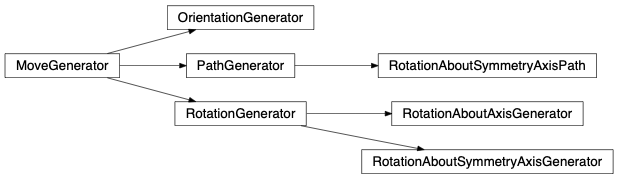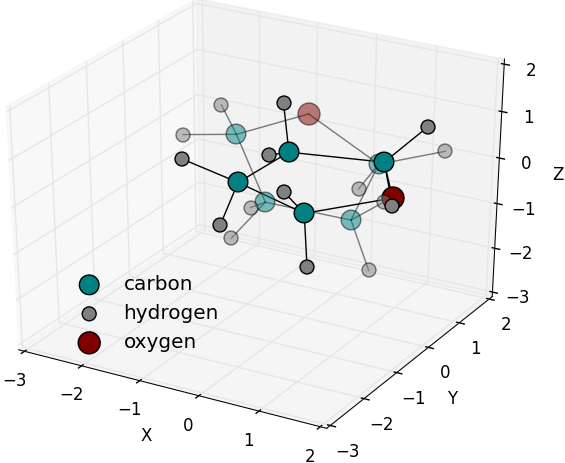Random rotation axis and angle generated and applied on a Tetrahydrofuran molecule. Solid colours are of the origin molecule position while fading ones are of the rotated molecule. (RotationGenerator)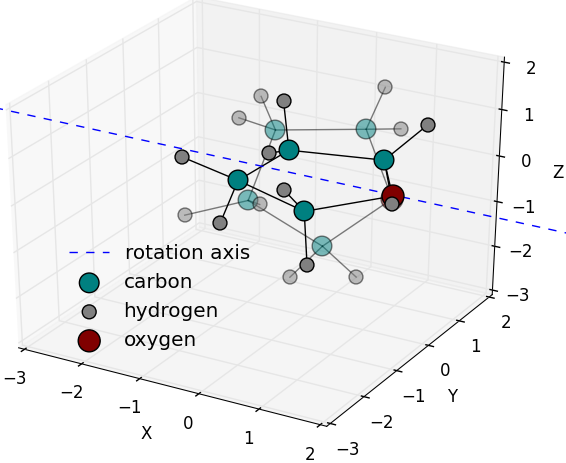Random rotation generated about a pre-defined axis or one of the symmetry axes of the Tetrahydrofuran molecule. Solid colours are of the origin molecule position while fading ones are of the rotated molecule. (RotationAboutAxisGenerator RotationAboutSymmetryAxisGenerator)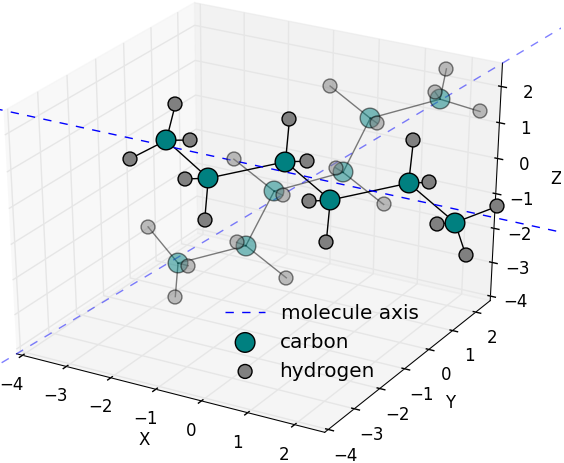Random orientation of hexane molecule to [1,1,1] axis with maximumOffsetAngle of 10 degrees is generated. First principal axis of hexane molecule is considered as groupAxis. Solid colors are of original molecule while fading ones are of the oriented one. (OrientationGenerator)
class fullrmc.Generators.Rotations.RotationGenerator(group=None, amplitude=2)

Generate random rotational moves upon groups of atoms. Only groups of more than one atom are accepted.

Parameters: group (None, Group): The group instance. amplitude (number): The maximum rotation angle allowed in degrees. It must be strictly bigger than 0 and strictly smaller than 360.
# import fullrmc modules
from fullrmc.Engine import Engine
from fullrmc.Generators.Rotations import RotationGenerator

# create engine
ENGINE = Engine(path='my_engine.rmc')

# set pdb file
ENGINE.set_pdb('system.pdb')

# Add constraints ...
# Re-define groups if needed ...
# Re-define groups selector if needed ...

# set moves generators to random rotations.
# Maximum rotation amplitude is set to 5 degrees to all defined groups
for g in ENGINE.groups:
if len(g) >1:
g.set_move_generator( RotationGenerator(amplitude=5) )

amplitude

Maximum allowed angle of rotation in rad.

set_amplitude(amplitude)

Set maximum rotation angle in degrees and transforms it to rad.

Parameters: amplitude (number): the maximum allowed rotation angle in degrees. It must be strictly bigger than 0 and strictly smaller than 360.
check_group(group)

Check the generator’s group.

Parameters: group (Group): the Group instance.
transform_coordinates(coordinates, argument=None)

Rotate coordinates.

Parameters: coordinates (np.ndarray): The coordinates on which to apply the rotation. argument (object): Any python object. Not used in this generator. coordinates (np.ndarray): The new coordinates after applying the rotation.
class fullrmc.Generators.Rotations.RotationAboutAxisGenerator(group=None, amplitude=2, axis=(1, 0, 0))

Generates random rotational moves upon groups of atoms about a pre-defined axis.

Parameters: group (None, Group): The group instance. amplitude (number): The maximum allowed rotation angle in degrees. It must be strictly bigger than 0 and strictly smaller than 360. axis (list,set,tuple,numpy.ndarray): The rotational axis vector.
# import fullrmc modules
from fullrmc.Engine import Engine
from fullrmc.Generators.Rotations import RotationAboutAxisGenerator

# create engine
ENGINE = Engine(path='my_engine.rmc')

# set pdb file
ENGINE.set_pdb('system.pdb')

# Add constraints ...
# Re-define groups if needed ...
# Re-define groups selector if needed ...

# set moves generators to random rotations about (1,1,1) a pre-defined axis.
# Maximum rotation amplitude is set to 5 degrees to all defined groups
for g in ENGINE.groups:
if len(g) >1:
g.set_move_generator( RotationAboutAxisGenerator(amplitude=5, axis=(1,1,1)) )

axis

Rotation axis vector.

check_group(group)

Check the generator’s group.

Parameters: group (Group): The Group instance.
set_axis(axis)

Set the axis along which the rotation will be performed.

Parameters: axis (list,set,tuple,numpy.ndarray): The rotation axis vector.
transform_coordinates(coordinates, argument=None)

Rotate coordinates.

Parameters: coordinates (np.ndarray): The coordinates on which to apply the rotation. argument (object): Not used here. coordinates (np.ndarray): The new coordinates after applying the rotation.
class fullrmc.Generators.Rotations.RotationAboutSymmetryAxisGenerator(group=None, amplitude=2, axis=0)

Generates random rotational moves upon groups of atoms about one of their symmetry axis. Only groups of more than 1 atom are accepted.

Parameters: group (None, fullrmc.Engine): The constraint fullrmc engine. amplitude (number): Maximum rotation angle in degrees. It must be strictly bigger than 0 and strictly smaller than 360. axis (integer): Must be 0,1 or 2 for respectively the main, secondary or tertiary symmetry axis
# import fullrmc modules
from fullrmc.Engine import Engine
from fullrmc.Generators.Rotations import RotationAboutSymmetryAxisGenerator

# create engine
ENGINE = Engine(path='my_engine.rmc')

# set pdb file
ENGINE.set_pdb('system.pdb')

# Add constraints ...
# Re-define groups if needed ...
# Re-define groups selector if needed ...

# set moves generators to random rotations about the second symmetry axis of each group.
# Maximum rotation amplitude is set to 5 degrees to all defined groups
for g in ENGINE.groups:
if len(g) >1:
g.set_move_generator( RotationAboutSymmetryAxisGenerator(amplitude=5, axis=1) )

axis

Rotation axis index.

set_axis(axis)

Set the symmetry axis index to rotate about.

Parameters: axis (integer): Must be 0,1 or 2 for respectively the main, secondary or tertiary symmetry axis.
transform_coordinates(coordinates, argument=None)

Rotate coordinates.

Parameters: coordinates (np.ndarray): The coordinates on which to apply the rotation. argument (object): Not used here. coordinates (np.ndarray): The new coordinates after applying the rotation.
class fullrmc.Generators.Rotations.RotationAboutSymmetryAxisPath(group=None, axis=0, path=None, randomize=False)

Generate rotational moves upon groups of atoms about one of their symmetry axis. Only groups of more than one atom are accepted.

Parameters: group (None, fullrmc.Engine): The constraint fullrmc engine. axis (integer): Must be 0,1 or 2 for respectively the main, secondary or tertiary symmetry axis. path (List): list of angles. randomize (boolean): Whether to pull moves randomly from path or pull moves in order at every step.
# import fullrmc modules
from fullrmc.Engine import Engine
from fullrmc.Generators.Rotations import RotationAboutSymmetryAxisPath

# create engine
ENGINE = Engine(path='my_engine.rmc')

# set pdb file
ENGINE.set_pdb('system.pdb')

# Add constraints ...
# Re-define groups if needed ...
# Re-define groups selector if needed ...

# set moves generators to pre-defined rotations about the second symmetry axis of each group.
angles = [-0.1, -0.5, -0.05, 0.5, 0.01, 2, 3, 1, -3]
for g in ENGINE.groups:
if len(g) >1:
g.set_move_generator( RotationAboutSymmetryAxisPath(axis=1, path=angles) )

axis

Rotation axis index.

set_axis(axis)

Set the symmetry axis index to rotate about.

Parameters: axis (integer): Must be 0,1 or 2 for respectively the main, secondary or tertiary symmetry axis
check_path(path)

Check the generator’s path.

Parameters: path (None, list): The list of moves.
normalize_path(path)

Transform all path angles to radian.

Parameters: path (list): The list of moves in degrees. path (list): The list of moves in rad.
check_group(group)

Check the generator’s group.

Parameters: group (Group): The Group instance.
transform_coordinates(coordinates, argument)

Rotate coordinates.

Parameters: coordinates (np.ndarray): The coordinates on which to apply the rotation. argument (object): The rotation angle. coordinates (np.ndarray): The new coordinates after applying the rotation.
class fullrmc.Generators.Rotations.OrientationGenerator(group=None, maximumOffsetAngle=10, groupAxis={'symmetry': 0}, orientationAxis={'fixed': (1, 0, 0)}, flip=None)

Generate rotational moves upon groups of atoms to align and orient along an axis. Orientation rotations are computed randomly allowing offset angle between grouAxis and orientationAxis Only groups of more than 1 atom are accepted.

Parameters: group (None, Group): The group instance. maximumOffsetAngle (number): The maximum offset angle in degrees between groupAxis and orientationAxis. groupAxis (dict): The group axis. Only one key is allowed. If key is ‘fixed’, value must be a list, tuple or a numpy.array of a vector such as [X,Y,Z]. If key is ‘symmetry’, in this case the group axis is computed as one of the three symmetry axis of the group atoms. the value must be even 0, 1 or 2 for respectively the first, second and tertiary symmetry axis. orientationAxis (dict): The axis to align the group with. If key is ‘fixed’, value must be a list, tuple or a numpy.array of a vector such as [X,Y,Z]. If Key is ‘symmetry’, in this case the value must be a list of two items, the first one is a list of atoms indexes to compute symmetry axis and the second item must be even 0, 1 or 2 for respectively the first, second and tertiary symmetry axis. flip (None, bool): Whether to allow flipping axis orientation or not. If True, orientationAxis will be flipped forcing anti-parallel orientation. If False, orientationAxis will not be flipped forcing parallel orientation. If None is given, no flipping is forced, flipping can be set randomly to True or False during run time execution.
# import fullrmc modules
from fullrmc.Engine import Engine
from fullrmc.Generators.Rotations import OrientationGenerator

# create engine
ENGINE = Engine(path='my_engine.rmc')

# set pdb file
ENGINE.set_pdb('system.pdb')

# Add constraints ...
# Re-define groups if needed ...
# Re-define groups selector if needed ...

# set moves generators to orientations of each group third symmetry axis
# towards the (-1,0,2) the predefined axis within maximum 5 degrees.
for g in ENGINE.groups:
if len(g) >1:
g.set_move_generator( OrientationGenerator(maximumOffsetAngle=5,
groupAxis={"symmetry":2},
orientationAxis={"fixed":(-1,0,2)}) )

maximumOffsetAngle

Maximum offset angle in degrees between groupAxis and orientationAxis in rad.

orientationAxis

Orientation axis value or definition.

groupAxis

Group axis value or definition.

flip

Flip value.

set_maximum_offset_angle(maximumOffsetAngle)

Set the maximum offset angle allowed.

Parameters: maximumOffsetAngle (number): The maximum offset angle in degrees between groupAxis and orientationAxis.
check_group(group)

Check the generator’s group.

Parameters: group (Group): the Group instance.
set_flip(flip)

Set flip flag value.

Parameters: flip (None, bool): Whether to allow flipping axis orientation or not. If True, orientationAxis will be flipped forcing anti-parallel orientation. If False, orientationAxis will not be flipped forcing parallel orientation. If None is given, no flipping is forced, flipping can be set randomly to True or False during run time execution.
set_group_axis(groupAxis)

Sets group axis value.

Parameters: groupAxis (dict): The group axis. Only one key is allowed. If key is fixed, value must be a list, tuple or a numpy.array of a vector such as [X,Y,Z]. If key is symmetry, in this case the group axis is computed as one of the three symmetry axis of the group atoms. the value must be even 0, 1 or 2 for respectively the first, second and tertiary symmetry axis.
set_orientation_axis(orientationAxis)

Set orientation axis value.

Parameters: orientationAxis (dict): The axis to align the group axis with. If key is fixed, value must be a list, tuple or a numpy.array of a vector such as [X,Y,Z]. If Key is symmetry, in this case the value must be a list of two items, the first one is a list of atoms indexes to compute symmetry axis and the second item must be even 0, 1 or 2 for respectively the first, second and tertiary symmetry axis.
transform_coordinates(coordinates, argument=None)

Rotate coordinates.

Parameters: coordinates (np.ndarray): The coordinates on which to apply the rotation. argument (object): Not used here. coordinates (np.ndarray): The new coordinates after applying the rotation.
fullrmc.Generators.Rotations.generate_random_float()

random() -> x in the interval [0, 1).

## Translations¶

Translations contains all translation like MoveGenerator classes.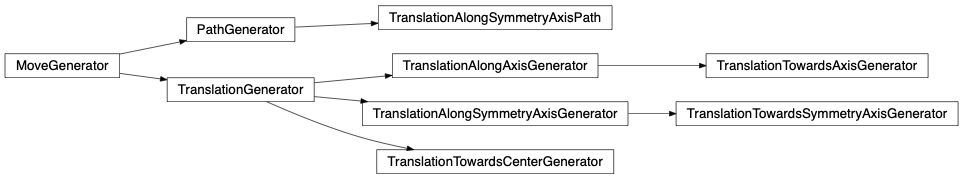Random translation vectors generated from atom at origin. (TranslationGenerator)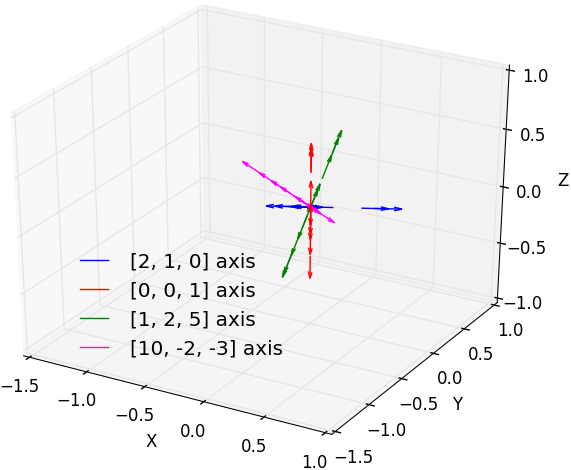Random translation vectors generated from atom at origin along a pre-defined axis. (TranslationAlongAxisGenerator)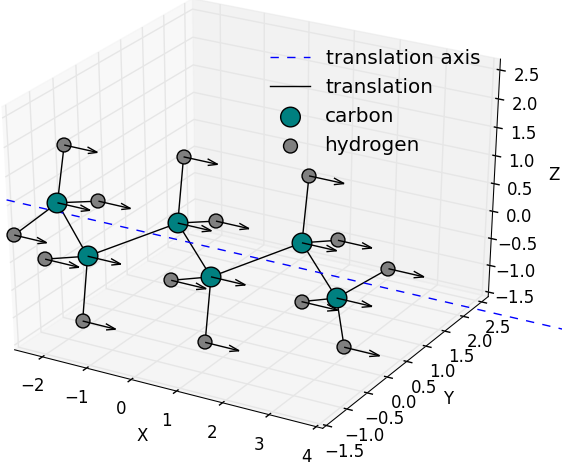Random translation vector generated along a predefined axis or one of the symmetry axes of the hexane molecule and applied on all the molecule’s atoms at the same time. (TranslationAlongAxisGenerator TranslationAlongSymmetryAxisGenerator)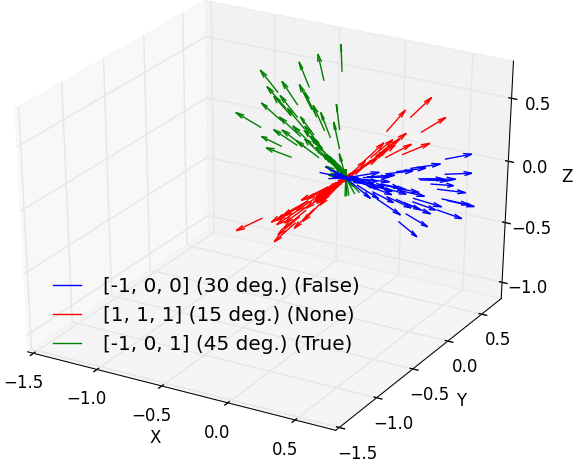Random translation vectors generated towards an axis within some maximum angle. Legend is formatted as axis (angle) (direction) (TranslationTowardsAxisGenerator TranslationTowardsSymmetryAxisGenerator)Random translation vectors generated towards a pre-defined center or towards the geometric center of a group of atoms. Here 20 vectors are generated within a maximum separation angle of 30 deg. (TranslationTowardsCenterGenerator)

class fullrmc.Generators.Translations.TranslationGenerator(group=None, amplitude=0.2)

Generates random translations moves upon groups of atoms.

Parameters: group (None, Group): The group instance. amplitude (number, tuple): The translation amplitude in Angstroms. If number is given, it is the maximum translation amplitude in Angstroms and must be bigger than 0. If tuple is given, it is the limits of translation boundaries as [min,max] where min>=0 and max>min.
# import fullrmc modules
from fullrmc.Engine import Engine
from fullrmc.Generators.Translations import TranslationGenerator

# create engine
ENGINE = Engine(path='my_engine.rmc')

# set pdb file
ENGINE.set_pdb('system.pdb')

# Add constraints ...
# Re-define groups if needed ...
# Re-define groups selector if needed ...

# set moves generators to random translations.
# Maximum translation amplitude is set to 0.3A to all defined groups
for g in ENGINE.groups:
g.set_move_generator( TranslationGenerator(amplitude=0.3) )

amplitude

Translation amplitude limits.

set_amplitude(amplitude)

Set maximum translation vector allowed amplitude.

Parameters: amplitude (number, tuple): The translation amplitude in Angstroms. If number is given, it is the maximum translation amplitude in Angstroms and must be bigger than 0. If tuple is given, it is the limits of translation boundaries as [min,max] where min>=0 and max>min.
check_group(group)

Check the generator’s group.

Parameters: group (Group): the Group instance.
transform_coordinates(coordinates, argument=None)

Translate coordinates.

Parameters: coordinates (np.ndarray): The coordinates on which to apply the translation. coordinates (np.ndarray): The new coordinates after applying the translation. argument (object): Any python object. Not used in this generator.
class fullrmc.Generators.Translations.TranslationAlongAxisGenerator(group=None, amplitude=0.2, axis=(1, 0, 0), direction=None)

Generates random translation moves upon groups of atoms along a pre-defined axis.

Parameters: group (None, fullrmc.Engine): The constraint stochastic engine. amplitude (number, tuple): The translation amplitude in Angstroms. If number is given, it is the maximum translation amplitude in Angstroms and must be bigger than 0. If tuple is given, it is the limits of translation boundaries as [min,max] where min>=0 and max>min. axis (list,set,tuple,numpy.ndarray): The pre-defined translation axis vector. direction (None, True, False): Whether to generate translation vector in the same direction of axis or not. If None is given, generated axis can be in the same direction of axis or in the opposite. If True is given, all generated vectors are in the same direction of axis. If False is given, all generated vectors are in the opposite direction of axis.
# import fullrmc modules
from fullrmc.Engine import Engine
from fullrmc.Generators.Translations import TranslationAlongAxisGenerator

# create engine
ENGINE = Engine(path='my_engine.rmc')

# set pdb file
ENGINE.set_pdb('system.pdb')

# Add constraints ...
# Re-define groups if needed ...
# Re-define groups selector if needed ...

# set moves generators to translations along pre-defined axis (1,1,1).
# Maximum translation amplitude is set to 0.3A to all defined groups
for g in ENGINE.groups:
g.set_move_generator( TranslationAlongAxisGenerator(amplitude=0.3, axis=(1,1,1)) )

axis

Translation axis.

direction

Generated translation vectors direction.

set_axis(axis)

Set the axis along which the translation will be performed.

Parameters: axis (list,set,tuple,numpy.ndarray): Translation axis vector.
set_direction(direction)

Sets the generated translation vectors direction.

Parameters: direction (None, True, False): Whether to generate translation vector in the same direction of axis or not. If None generated axis can be in the same direction of axis or in the opposite. If True all generated vectors are in the same direction of axis. If False all generated vectors are in the opposite direction of axis.
transform_coordinates(coordinates, argument=None)

translates coordinates.

Parameters: coordinates (np.ndarray): The coordinates on which to apply the translation. coordinates (np.ndarray): The new coordinates after applying the translation. argument (object): Any python object. Not used in this generator.
class fullrmc.Generators.Translations.TranslationTowardsAxisGenerator(group=None, amplitude=0.2, axis=(1, 0, 0), angle=30, direction=True)

Generates random translation moves upon groups of atoms towards a pre-defined axis within a tolerance angle between translation vectors and the pre-defined axis.

Parameters: group (None, fullrmc.Engine): The constraint stochastic engine. amplitude (number, tuple): The translation amplitude in Angstroms. If number is given, it is the maximum translation amplitude in Angstroms and must be bigger than 0. If tuple is given, it is the limits of translation boundaries as [min,max] where min>=0 and max>min. axis (list,set,tuple,numpy.ndarray): The pre-defined translation axis vector. angle (number): The maximum tolerance angle in degrees between a generated translation vector and the pre-defined axis. direction (None, True, False): Whether to generate translation vector in the same direction of axis or not. If None generated axis can be in the same direction of axis or in the opposite. If True all generated vectors are in the same direction of axis. If False all generated vectors are in the opposite direction of axis.
# import fullrmc modules
from fullrmc.Engine import Engine
from fullrmc.Generators.Translations import TranslationTowardsAxisGenerator

# create engine
ENGINE = Engine(path='my_engine.rmc')

# set pdb file
ENGINE.set_pdb('system.pdb')

# Add constraints ...
# Re-define groups if needed ...
# Re-define groups selector if needed ...

# set moves generators to translations towards a pre-defined axis (1,1,1) within 10 degrees.
# Maximum translation amplitude is set to 0.3A to all defined groups
for g in ENGINE.groups:
g.set_move_generator( TranslationTowardsAxisGenerator(amplitude=0.3, axis=(1,1,1), angle=10) )

angle

Tolerance maximum angle in rad.

set_angle(angle)

Set the tolerance maximum angle.

Parameters: angle (number): The maximum tolerance angle in degrees between a generated translation vector and the pre-defined axis.
transform_coordinates(coordinates, argument=None)

translate coordinates.

Parameters: coordinates (np.ndarray): The coordinates on which to apply the translation. coordinates (np.ndarray): The new coordinates after applying the translation. argument (object): Not used here.
class fullrmc.Generators.Translations.TranslationAlongSymmetryAxisGenerator(group=None, amplitude=0.2, axis=0, direction=None)

Generate random translation moves upon groups of atoms along one of their symmetry axis. Only groups containing more than 1 atoms allow computing symmetry axis.

Parameters: group (None, Group): The group instance. amplitude (number, tuple): The translation amplitude in Angstroms. If number is given, it is the maximum translation amplitude in Angstroms and must be bigger than 0. If tuple is given, it is the limits of translation boundaries as [min,max] where min>=0 and max>min. axis (integer): Must be 0,1 or 2 for respectively the mains, secondary or tertiary symmetry axis. direction (None, True, False): Whether to generate translation vector in the same direction of axis or not. If None generated axis can be in the same direction of axis or in the opposite. If True all generated vectors are in the same direction of axis. If False all generated vectors are in the opposite direction of axis.
# import fullrmc modules
from fullrmc.Engine import Engine
from fullrmc.Generators.Translations import TranslationAlongSymmetryAxisGenerator

# create engine
ENGINE = Engine(path='my_engine.rmc')

# set pdb file
ENGINE.set_pdb('system.pdb')

# Add constraints ...
# Re-define groups if needed ...
# Re-define groups selector if needed ...

# set moves generators to translations along the second symmetry axis of every group.
# Maximum translation amplitude is set to 0.3A to all defined groups.
for g in ENGINE.groups:
if len(g)>1:
g.set_move_generator( TranslationAlongSymmetryAxisGenerator(amplitude=0.3, axis=(1,1,1), axis=1) )

axis

Translation axis index.

direction

Generated translation vectors direction.

check_group(group)

Check the generator’s group.

Parameters: group (Group): the Group instance.
set_axis(axis)

Set the symmetry axis index to translate along.

Parameters: axis (integer): Must be 0,1 or 2 for respectively the main, secondary or tertiary symmetry axis
set_direction(direction)

Sets the generated translation vectors direction.

Parameters: direction (None, True, False): Whether to generate translation vector in the same direction of axis or not. If None generated axis can be in the same direction of axis or in the opposite. If True all generated vectors are in the same direction of axis. If False all generated vectors are in the opposite direction of axis.
transform_coordinates(coordinates, argument=None)

translate coordinates.

Parameters: coordinates (np.ndarray): The coordinates on which to apply the translation. coordinates (np.ndarray): The new coordinates after applying the translation. argument (object): Not used here.
class fullrmc.Generators.Translations.TranslationTowardsSymmetryAxisGenerator(group=None, amplitude=0.2, axis=0, angle=30, direction=True)

Generates random translation moves upon groups of atoms towards one of its symmetry axis within a tolerance angle between translation vectors and the axis. Only groups of more than 1 atom are accepted.

Parameters: group (None, fullrmc.Engine): The constraint stochastic engine. amplitude (number, tuple): The translation amplitude in Angstroms. If number is given, it is the maximum translation amplitude in Angstroms and must be bigger than 0. If tuple is given, it is the limits of translation boundaries as [min,max] where min>=0 and max>min. axis (integer): Must be 0,1 or 2 for respectively the mains, secondary or tertiary symmetry axis. angle (number): The maximum tolerance angle in degrees between a generated translation vector and the pre-defined axis. direction (None, True, False): Whether to generate translation vector in the same direction of axis or not. If None generated axis can be in the same direction of axis or in the opposite. If True all generated vectors are in the same direction of axis. If False all generated vectors are in the opposite direction of axis.
# import fullrmc modules
from fullrmc.Engine import Engine
from fullrmc.Generators.Translations import TranslationTowardsSymmetryAxisGenerator

# create engine
ENGINE = Engine(path='my_engine.rmc')

# set pdb file
ENGINE.set_pdb('system.pdb')

# Add constraints ...
# Re-define groups if needed ...
# Re-define groups selector if needed ...

# set moves generators to translations towards the first symmetry axis of every group within 15 degrees.
# Maximum translation amplitude is set to 0.3A to all defined groups.
for g in ENGINE.groups:
if len(g)>1:
g.set_move_generator( TranslationTowardsSymmetryAxisGenerator(amplitude=0.3, axis=0, angle=15) )

angle

Tolerance maximum angle in rad.

set_angle(angle)

Set the tolerance maximum angle.

Parameters: angle (number): The maximum tolerance angle in degrees between a generated translation vector and the pre-defined axis.
transform_coordinates(coordinates, argument=None)

translate coordinates.

Parameters: coordinates (np.ndarray): The coordinates on which to apply the translation. coordinates (np.ndarray): The new coordinates after applying the translation. argument (object): Not used here.
class fullrmc.Generators.Translations.TranslationAlongSymmetryAxisPath(group=None, axis=0, path=None, randomize=False)

Generates translation moves upon groups of atoms along one of their symmetry axis. Only groups of more than 1 atom are accepted.

Parameters: group (None, Group): The group instance. axis (integer): Must be 0,1 or 2 for respectively the main, secondary or tertiary symmetry axis path (List): List of distances. randomize (boolean): Whether to pull moves randomly from path or pull moves in order at every step.
# import fullrmc modules
from fullrmc.Engine import Engine
from fullrmc.Generators.Translations import TranslationAlongSymmetryAxisPath

# create engine
ENGINE = Engine(path='my_engine.rmc')

# set pdb file
ENGINE.set_pdb('system.pdb')

# Add constraints ...
# Re-define groups if needed ...
# Re-define groups selector if needed ...

# set moves generators to translations of predefined amplitudes along the first symmetry axis of every group.
amps = [-0.1, 0.075, -0.05, -0.25, 0.01, 0.02, 0.03, 0.1, 0.3]
for g in ENGINE.groups:
if len(g)>1:
g.set_move_generator( TranslationAlongSymmetryAxisPath(axis=0, path=amps) )

axis

Translation axis index.

check_group(group)

Check the generator’s group.

Parameters: group (Group): The Group instance.
set_axis(axis)

Set the symmetry axis index to translate along.

Parameters: axis (integer): Must be 0,1 or 2 for respectively the main, secondary or tertiary symmetry axis
check_path(path)

Check the generator’s path.

Parameters: path (None, list): The list of moves.
normalize_path(path)

Transforms all path distances to floating numbers.

Parameters: path (list): The list of moves. path (list): The list of moves.
transform_coordinates(coordinates, argument)

Translate coordinates.

Parameters: coordinates (np.ndarray): The coordinates on which to apply the translation. coordinates (np.ndarray): The new coordinates after applying the translation. argument (float): The move distance.
class fullrmc.Generators.Translations.TranslationTowardsCenterGenerator(group=None, center={'fixed': (0, 0, 0)}, amplitude=0.1, angle=30, direction=True)

Generates random translation moves of every atom of the group along its direction vector to the geometric center of the group.

Parameters: group (None, Group): The group instance. center (dict): The center value dictionary. Must have a single key and this can only be ‘fixed’ or ‘indexes’. If key is fixed, value must be a list or a numpy.array of a point coordinates such as [X,Y,Z]. If key is indexes, value must be a list or a numpy array of indexes. amplitude (number, tuple): The translation amplitude in Angstroms. If number is given, it is the maximum translation amplitude in Angstroms and must be bigger than 0. If tuple is given, it is the limits of translation boundaries as [min,max] where min>=0 and max>min. angle (None, number): The maximum tolerance angle in degrees between a generated translation vector and the computed direction. If None is given, all generated translation vectors will be along the direction to center. direction (None, True, False): Whether to generate translation vectors pointing towards the center or not. If None generated axis can be randomly generated towards the center or away from the center. If True is given, all generated vectors point towards the center. If False is given, all generated vectors point away from the center.
# import fullrmc modules
from fullrmc.Engine import Engine
from fullrmc.Generators.Translations import TranslationTowardsCenterGenerator

# create engine
ENGINE = Engine(path='my_engine.rmc')

# set pdb file
ENGINE.set_pdb('system.pdb')

# Add constraints ...
# Re-define groups if needed ...
# Re-define groups selector if needed ...

# set moves generators to translations towards the origin defined as (0,0,0) within 20 degrees.
# Maximum translation amplitude is set to 0.2A to all defined groups.
for g in ENGINE.groups:
g.set_move_generator( TranslationTowardsCenterGenerator(amplitude=0.2, center={"fixed":(0,0,0)}, axis=0, angle=25) )

direction

Direction value.

center

Center value.

angle

Angle value in rad.

set_direction(direction)

Set the generated translation vectors direction.

Parameters: direction (None, True, False): Whether to generate translation vector in the same direction of axis or not. If None generated axis can be in the same direction of axis or in the opposite. If True is given, all generated vectors are in the same direction of axis. If False is given, all generated vectors are in the opposite direction of axis.
set_angle(angle)

Sets the tolerance maximum angle.

Parameters: angle (None, number): The maximum tolerance angle in degrees between a generated translation vector and the computed direction. If None is given, all generated translation vectors will be along the direction to center.
set_center(center)

Set center value.

Parameters: center (dict): The center value dictionary. Must have a single key and this can only be ‘fixed’ or ‘indexes’. If key is fixed, value must be a list or a numpy.array of a point coordinates such as [X,Y,Z]. If key is indexes, value must be a list or a numpy array of indexes.
transform_coordinates(coordinates, argument=None)

Translate coordinates.

Parameters: coordinates (np.ndarray): The coordinates on which to apply the translation. coordinates (np.ndarray): The new coordinates after applying the translation. argument (object): Not used here.
fullrmc.Generators.Translations.generate_random_float()

random() -> x in the interval [0, 1).

## Agitations¶

Agitations contains all MoveGenerator classes that agitate and shake structures such as distances, angles, etc.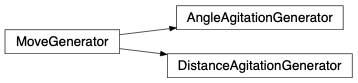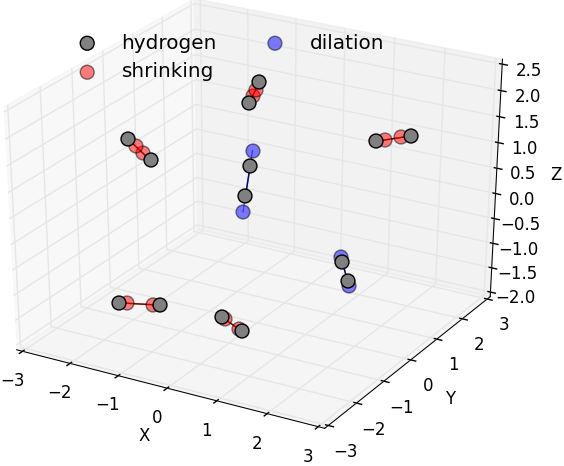Random H-H bond length agitations generated on dihydrogen molecules. At room temperature, H2 molecule bond length fluctuates around 0.74 Angstroms. Red hydrogen atoms represent the shrank H-H bond length molecule while blue hydrogen atoms represent the expanded H-H bond length molecules.Random H-O-H angle agitation generated on water molecules. At room temperature, water molecule angle formed between the two vectors formed between consecutively the Oxygen atom and the two hydrogen atoms is about 105 deg. Shrank H-O-H angles are represented by the red hydrogen while expanded angles are represented in blue. (AngleAgitationGenerator)
class fullrmc.Generators.Agitations.DistanceAgitationGenerator(group=None, amplitude=0.2, symmetric=True, shrink=None, agitate=(True, True))

Generates random agitation moves upon a distance separating two atoms by translating both atoms away from each other or closer to each other along the direction line between them. This is mainly used to shake two atoms bond distance by increasing and decreasing the bond length. Only groups of length 2 are accepted.

Parameters: group (None, Group): The group instance. It must contain exactly two indexes. amplitude (number): The maximum translation amplitude in Angstroms applied on every atom. symmetric (bool): Whether to apply the same amplitude of translation on both atoms or not. shrink (None, bool): Whether to always shrink the distance or expand it. If True, moves will always bring atoms closer to each other. If False, moves will always bring atoms away from each other. If None, no orientation is forced, therefore atoms can randomly get closer to each other or away from each other. agitate (tuple): It’s a tuple of two boolean values, at least one of them must be True. Whether to agitate the first atom, the second or both. This is useful to set an atom fixed while only the other succumb the agitation to adjust the distance. For instance in a C-H group it can be useful and logical to adjust the bond length by moving only the hydrogen atom along the bond direction.
# import fullrmc modules
from fullrmc.Engine import Engine
from fullrmc.Generators.Agitations import DistanceAgitationGenerator

# create engine
ENGINE = Engine(path='my_engine.rmc')

# set pdb file
ENGINE.set_pdb('system.pdb')

# Add constraints ...
# Re-define groups if needed ...
# Re-define groups selector if needed ...

# set moves generators to random agitations of distance seperating two atoms.
# Maximum agitation amplitude is set to to 0.5A
for g in ENGINE.groups:
if len(g)==2:
g.set_move_generator( DistanceAgitationGenerator(amplitude=0.5) )

amplitude

Maximum agitation amplitude.

shrink

Shrink flag value.

symmetric

Symmetric flag value.

agitate

Agitate tuple flags value.

check_group(group)

Check the generator’s group.

Parameters: group (Group): the Group instance.
set_amplitude(amplitude)

Sets maximum translation vector allowed amplitude.

Parameters: amplitude (number): the maximum allowed translation vector amplitude.
set_symmetric(symmetric)

Set symmetric flag value.

Parameters: symmetric (bool): Whether to apply the same amplitude of translation on both atoms or not.
set_shrink(shrink)

Set shrink flag value.

Parameters: shrink (None, bool): Whether to always shrink the distance or expand it. If True, moves will always bring atoms closer to each other. If False, moves will always bring atoms away from each other. If None, no orientation is forced, therefore distance can increase or decrease randomly at every step.
set_agitate(agitate)

Set agitate tuple value.

Parameters: agitate (tuple): It’s a tuple of two boolean values, at least one of them must be True. Whether to agitate the first atom, the second or both. This is useful to set an atom fixed while only the other succumb the agitation to adjust the distance. For instance in a C-H group it can be useful and logical to adjust the bond length by moving only the hydrogen atom along the bond direction.
transform_coordinates(coordinates, argument=None)

Translate coordinates.

Parameters: coordinates (np.ndarray): The coordinates on which to apply the translation. coordinates (np.ndarray): The new coordinates after applying the translation. argument (object): Any python object. Not used in this generator.
class fullrmc.Generators.Agitations.AngleAgitationGenerator(group=None, amplitude=2, symmetric=True, shrink=None, agitate=(True, True))

Generate random agitation moves upon an angle defined between two vectors left-central and right-central where (central, left, right) are three atoms. Move will be performed on left and/or right atom while central atom will always remain fixed. Distances between left/right and central atoms will remain unchanged. This is mainly used to shake bonded atoms angles by increasing and decreasing the bond length. Only groups of length 3 are accepted.

Parameters: group (None, Group): The group instance. It must contain exactly three indexes in respective order (central, left, right) atoms index. amplitude (number): The maximum agitation angle amplitude in degrees of left and right atoms separately. symmetric (bool): Whether to apply the same amplitude of rotation on both left and right atoms or not. shrink (None, bool): Whether to always shrink the angle or expand it. If True, moves will always reduce angle. If False, moves will always increase angle. If None, no orientation is forced, therefore angle can randomly get wider or tighter. agitate (tuple): It’s a tuple of two boolean values for respectively (left, right) atoms, at least one of them must be True. Whether to agitate the left atom, the right or both. This is useful to set an atom fixed while only the other succumb the agitation to adjust the angle.
# import fullrmc modules
from fullrmc.Engine import Engine
from fullrmc.Generators.Agitations import AngleAgitationGenerator

# create engine
ENGINE = Engine(path='my_engine.rmc')

# set pdb file
ENGINE.set_pdb('system.pdb')

# Add constraints ...
# Re-define groups if needed ...
# Re-define groups selector if needed ...

# set moves generators to random agitations of the angle formed between
# one central atom and other two. Maximum agitation amplitude is set to to 10.
for g in ENGINE.groups:
if len(g)==3:
g.set_move_generator( AngleAgitationGenerator(amplitude=10) )

amplitude

Maximum agitation angle amplitude in rad.

shrink

Shrink flag value.

symmetric

Symmetric flag value.

agitate

Agitate tuple flags value.

check_group(group)

Check the generator’s group.

Parameters: group (Group): the Group instance.
set_amplitude(amplitude)

Set maximum allowed agitation rotation angle amplitude in degrees of left and right atoms separately and transforms it to rad.

Parameters: amplitude (number): The maximum agitation angle amplitude in degrees of left and right atoms separately.
set_symmetric(symmetric)

Set symmetric flag value.

Parameters: symmetric (bool): Whether to apply the same amplitude of translation on both atoms or not.
set_shrink(shrink)

Set shrink flag value.

Parameters: shrink (None, bool): Whether to always shrink the distance or expand it. If True, moves will always bring atoms closer to each other. If False, moves will always bring atoms away from each other. If None, no orientation is forced, therefore distance can increase or decrease randomly at every step.
set_agitate(agitate)

Set agitate tuple value.

Parameters: agitate (tuple): It’s a tuple of two boolean values, at least one of them must be True. Whether to agitate the first atom, the second or both. This is useful to set an atom fixed while only the other succumb the agitation to adjust the distance. For instance in a C-H group it can be useful and logical to adjust the bond length by moving only the hydrogen atom along the bond direction.
transform_coordinates(coordinates, argument=None)

Translate coordinates.

Parameters: coordinates (np.ndarray): The coordinates on which to apply the translation. coordinates (np.ndarray): The new coordinates after applying the translation. argument (object): Any python object. Not used in this generator.
fullrmc.Generators.Agitations.generate_random_float()

random() -> x in the interval [0, 1).

## Swap¶

Swaps contains all swap or atoms position exchange MoveGenerator classes.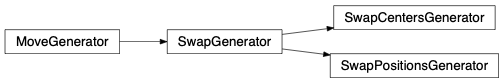class fullrmc.Generators.Swaps.SwapPositionsGenerator(group=None, swapLength=1, swapList=None)

Generates positional swapping between atoms of the selected group and other atoms randomly selected from swapList.

Parameters: group (None, Group): The group instance. swapLength (Integer): The swap length that defines the length of the group and the length of the every swap sub-list in swapList. swapList (None, List): The list of atoms. If None is given, no swapping or exchanging will be performed. If List is given, it must contain lists of atoms where every sub-list must have the same number of atoms as the group.
# import fullrmc modules
from fullrmc.Engine import Engine
from fullrmc.Generators.Swaps import SwapPositionsGenerator

# create engine
ENGINE = Engine(path='my_engine.rmc')

# set pdb file
ENGINE.set_pdb('system.pdb')

# Add constraints ...
# Re-define groups if needed ...
# Re-define groups selector if needed ...

##### set swap moves between Lithium and Manganese atoms in Li2MnO3 system #####
# reset engine groups to atoms to insure atomic grouping of all the system's atoms
ENGINE.set_groups_as_atoms()
# get all elements list
elements = ENGINE.allElements
# create list of lithium atoms indexes
liIndexes = [[idx] for idx in xrange(len(elements)) if elements[idx]=='li']
# create list of manganese atoms indexes
mnIndexes = [[idx] for idx in xrange(len(elements)) if elements[idx]=='mn']
# create swap generator to lithium atoms
swapWithLi = SwapPositionsGenerator(swapList=liIndexes)
# create swap generator to manganese atoms
swapWithMn = SwapPositionsGenerator(swapList=mnIndexes)
# set swap generator to groups
for g in ENGINE.groups:
# get group's atom index
idx = g.indexes
# set swap to manganese for lithium atoms
if elements[idx]=='li':
g.set_move_generator(swapWithMn)
# set swap to lithium for manganese atoms
elif elements[idx]=='mn':
g.set_move_generator(swapWithLi)
# the rest are oxygen atoms. Default RandomTranslation generator are kept.

check_group(group)

Check the generator’s group.

Parameters: group (Group): The Group instance.
set_swap_length(swapLength)

Set swap length. It will reset swaplist automatically.

Parameters: swapLength (Integer): The swap length that defines the length of the group and the length of the every swap sub-list in swapList.
transform_coordinates(coordinates, argument=None)

Transform coordinates by swapping. This method is called in every move.

Parameters: coordinates (np.ndarray): The coordinates on which to apply the swapping. argument (object): Any other argument needed to perform the move. In General it’s not needed. coordinates (np.ndarray): The new coordinates after applying the move.
class fullrmc.Generators.Swaps.SwapCentersGenerator(group=None, swapList=None)

Computes geometric center of the selected group, and swaps its atoms by translation to the atoms geometric center of the other atoms which are randomly selected from swapList and vice-versa.

Parameters: group (None, Group): The group instance. swapList (None, List): The list of atoms. If None is given, no swapping or exchanging will be performed. If List is given, it must contain lists of atoms index.
# import fullrmc modules
from fullrmc.Engine import Engine
from fullrmc.Generators.Swaps import SwapCentersGenerator

# create engine
ENGINE = Engine(path='my_engine.rmc')

# set pdb file
ENGINE.set_pdb('system.pdb')

# Add constraints ...
# Re-define groups if needed ...
# Re-define groups selector if needed ...

##### set swap moves between first 10 molecular groups of a system #####
# reset engine groups to molecules
ENGINE.set_groups_as_molecules()
# set swap generator to the first 10 groups
GROUPS = [ENGINE.groups[idx] for idx in range(10)]
for gidx, group in enumerate(GROUPS):
swapList = [g.indexes for idx,g in enumerate(GROUPS) if idx!=gidx]
swapGen  = SwapCentersGenerator(swapList=swapList)
group.set_move_generator(swapGen)

swapLength

Swap length. In this Case it is always None as swapLength is not required for this generator.

set_swap_length(swapLength)

Set swap length. The swap length that defines the length of the group and the length of the every swap sub-list in swapList. It will automatically be set to None as SwapCentersGenerator does not require a fixed length.

Parameters: swapLength (None): The swap length.
set_group(group)

Set the MoveGenerator group.

Parameters: group (None, Group): group instance.
check_group(group)

Check the generator’s group.

Parameters: group (Group): the Group instance.
transform_coordinates(coordinates, argument=None)

Transform coordinates by swapping. This method is called in every move.

Parameters: coordinates (np.ndarray): The coordinates on which to apply the swapping. argument (object): Any other argument needed to perform the move. In General it’s not needed. coordinates (np.ndarray): The new coordinates after applying the move.

## Removes¶

class fullrmc.Generators.Removes.AtomsRemoveGenerator(group=None, maximumCollected=None, atomsList=None)

This generator allows removing single atoms at a time from the system. Atoms are randomly picked from atomsList and returned upon calling pick_from_list method.

Parameters: group (None, Group): The group instance which is this case must be fullrmc EmptyGroup. maximumCollected (None, Integer): The maximum number allowed of atoms to be removed and collected from the engine. This property is general to the system and checks engine’s collected atoms not the number of removed atoms via this generator. If None is given, the remover will not check for the number of already removed atoms before attempting a remove. atomsList (None, list,set,tuple,np.ndarray): The list of atoms index to chose and remove from. If None is given, then all atoms in system will be used.
pick_from_list(engine)

Randomly picks an atom’s index from atomsList. This method checks and verifies maximumCollected prior to picking and index.

Parameters: engine (Engine): The engine calling the method. index (None, np.ndarray): Atom index wrapped in a numpy.ndarray. If None is returned than picking is not allowed.